# Selina Solutions Concise Mathematics Class 6 Chapter 10 Sets

Selina Solutions Concise Mathematics Class 6 Chapter 10 Sets helps students to be familiar with different types of questions that would appear in exams. Students can make use of Selina Solutions in solving exercise wise problems accurately presented in every chapter. Selina Solutions are formulated by the expert teachers in accordance with ICSE guidelines, to help students in boosting exam preparation.

A collection of well defined objects is known as Sets. Some of the important topics covered in this Chapter are elements of a Set, properties of a Set and notation of a Set. By following this Chapter, students can understand the fundamental concepts covered in Sets thoroughly. Students can access Selina Solutions Concise Mathematics Class 6 Chapter 10 Sets in PDF format, from the links given below

## Selina Solutions Concise Mathematics Class 6 Chapter 10: Sets Download PDF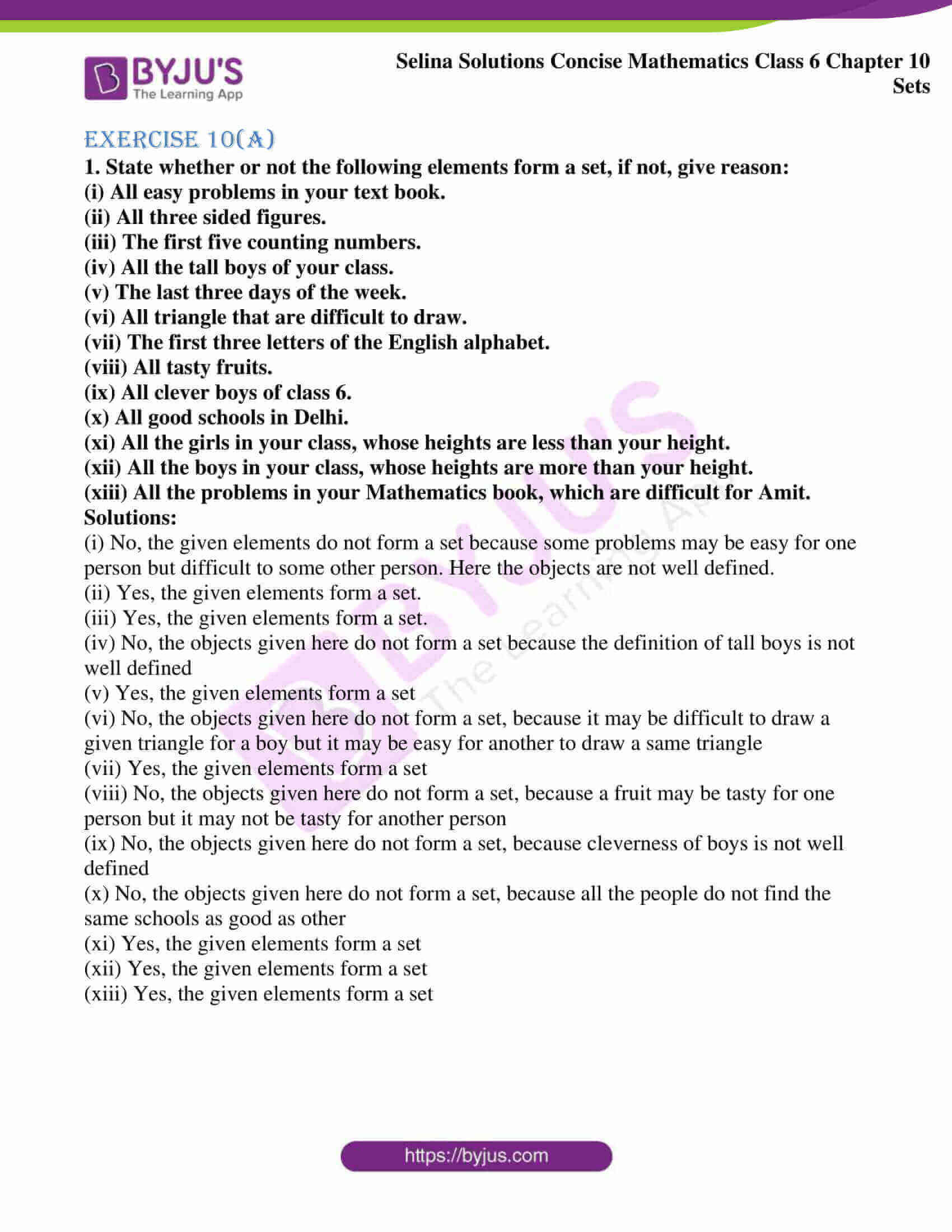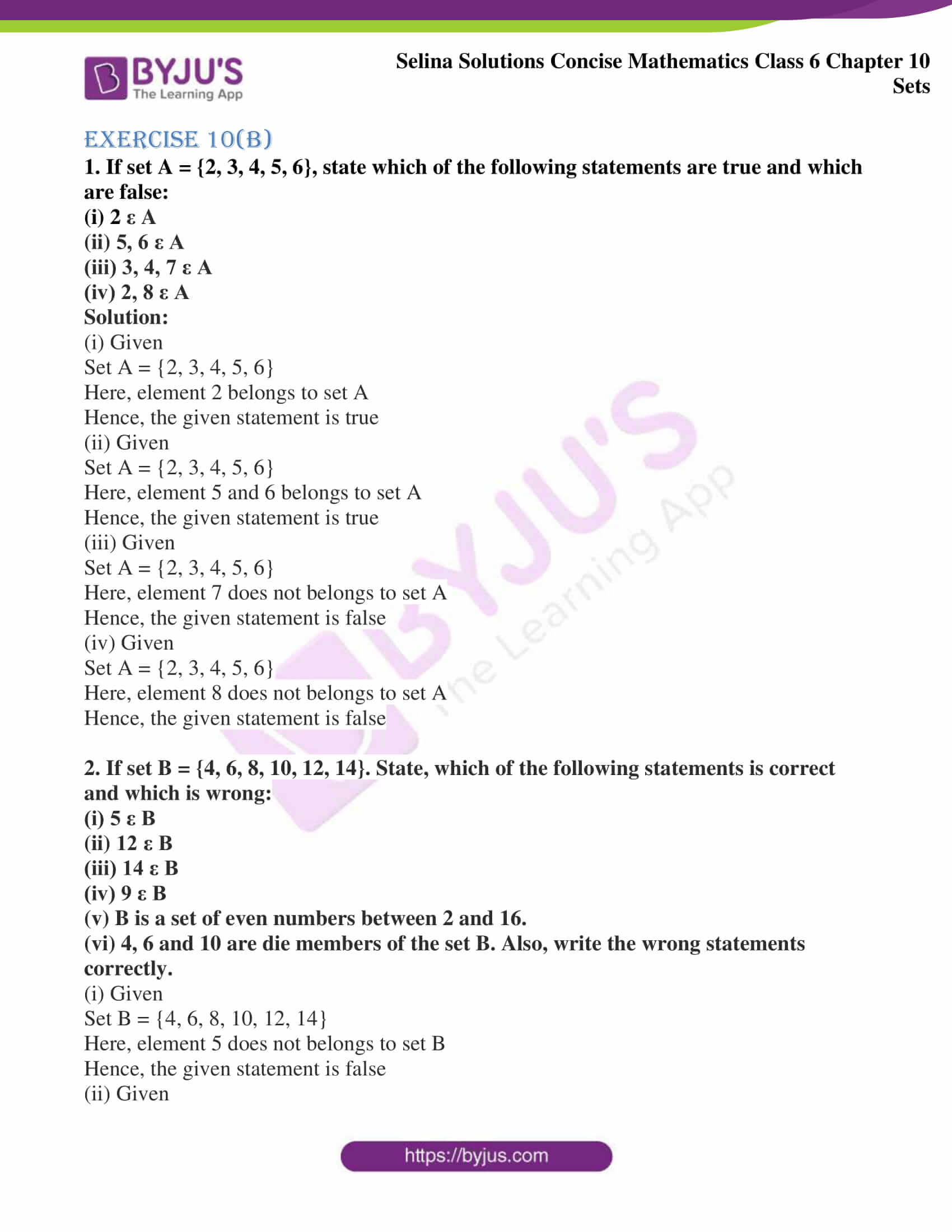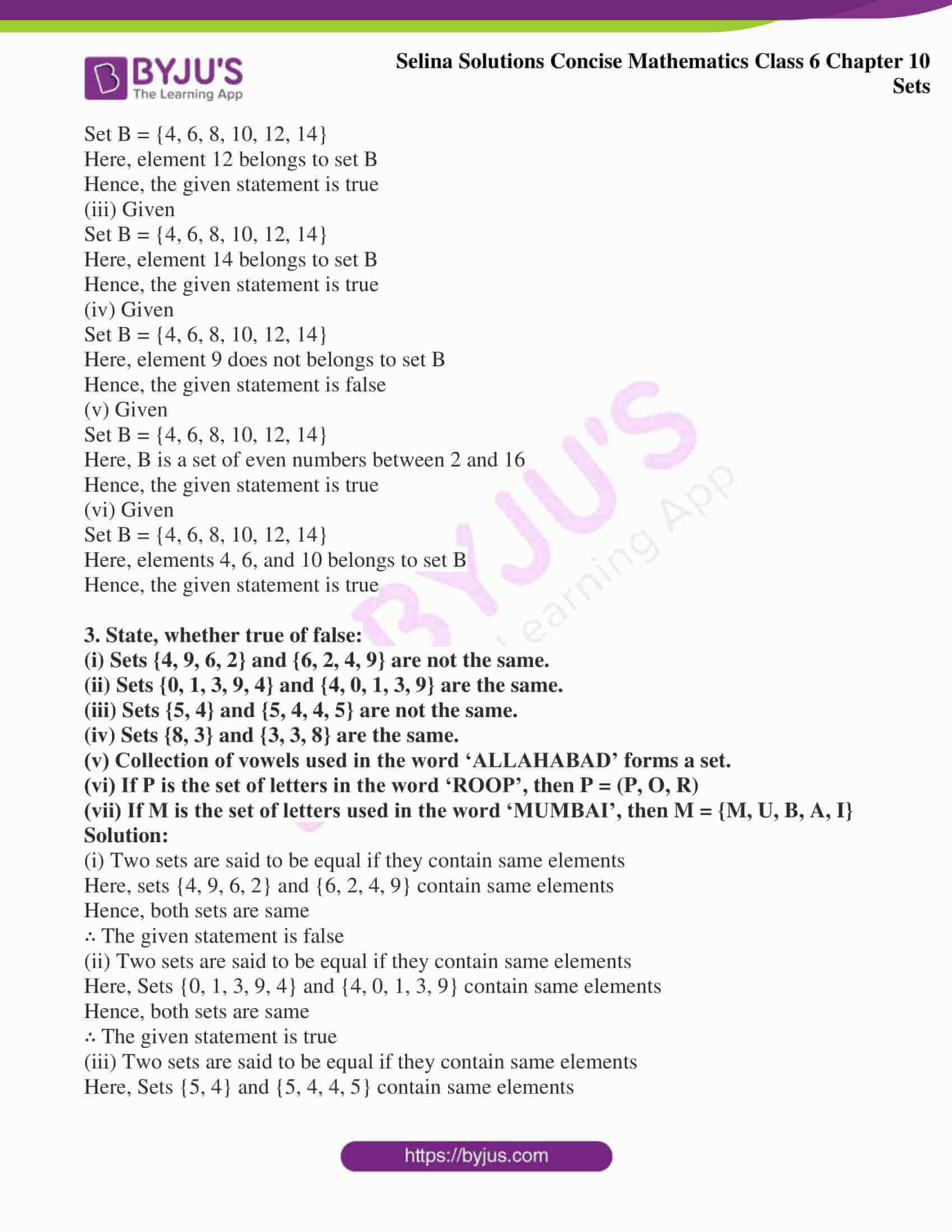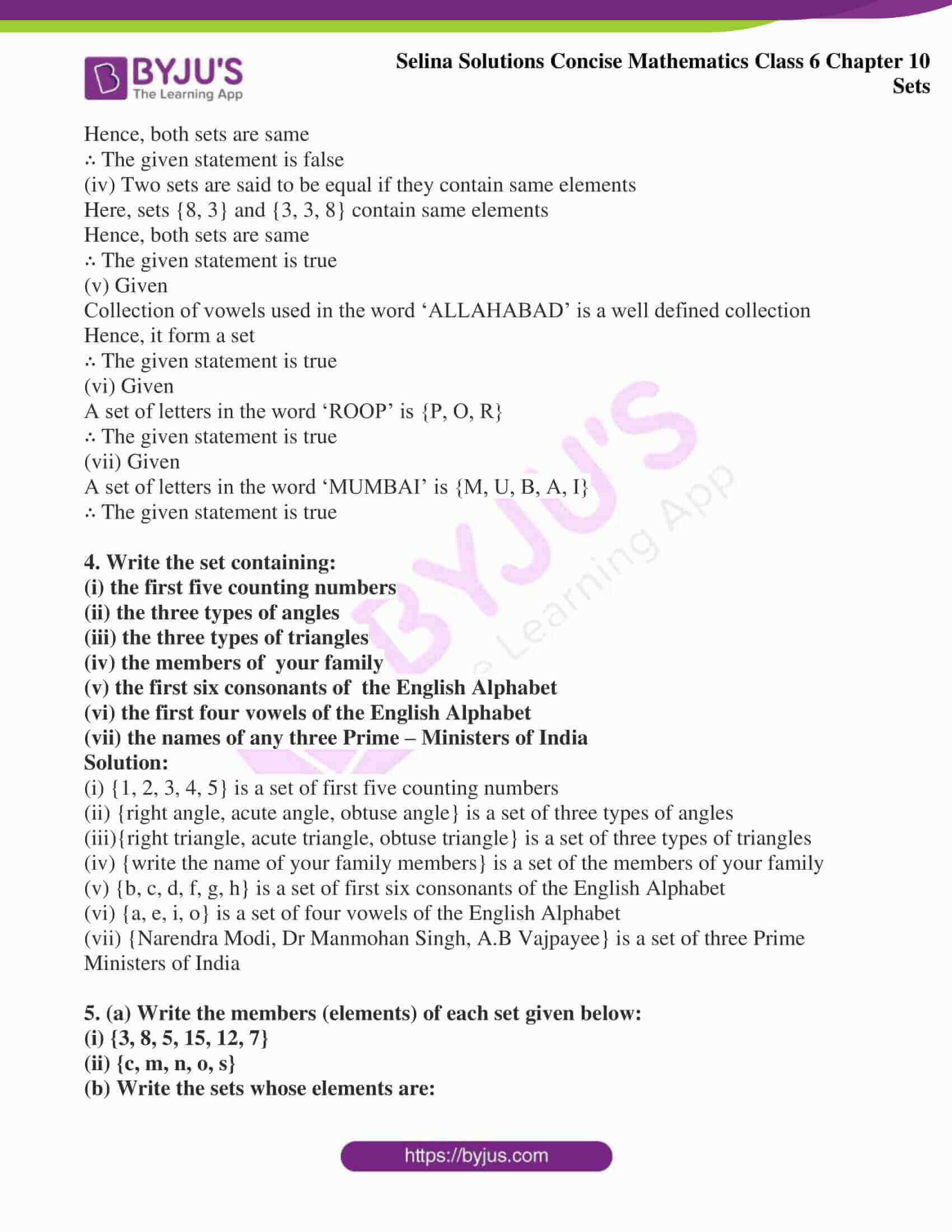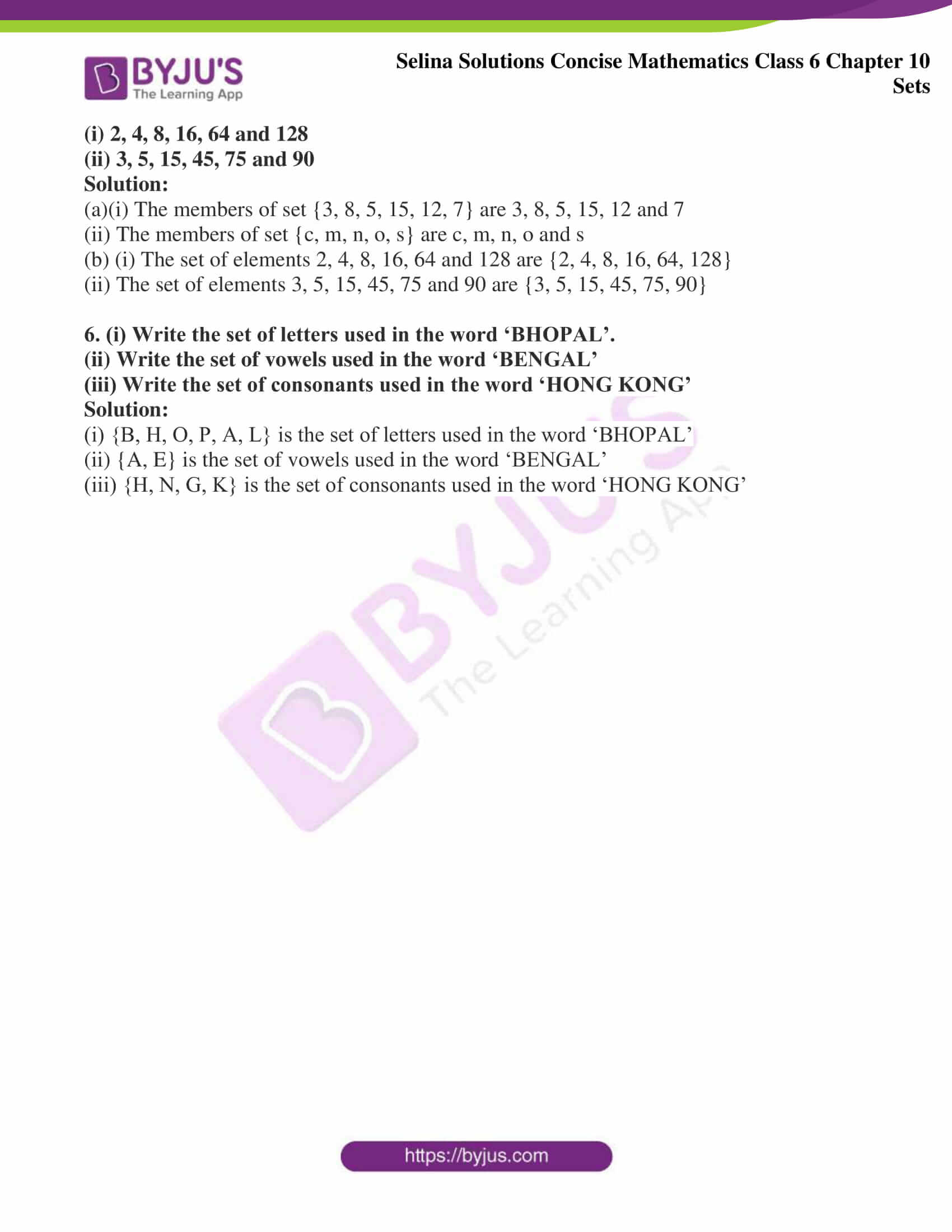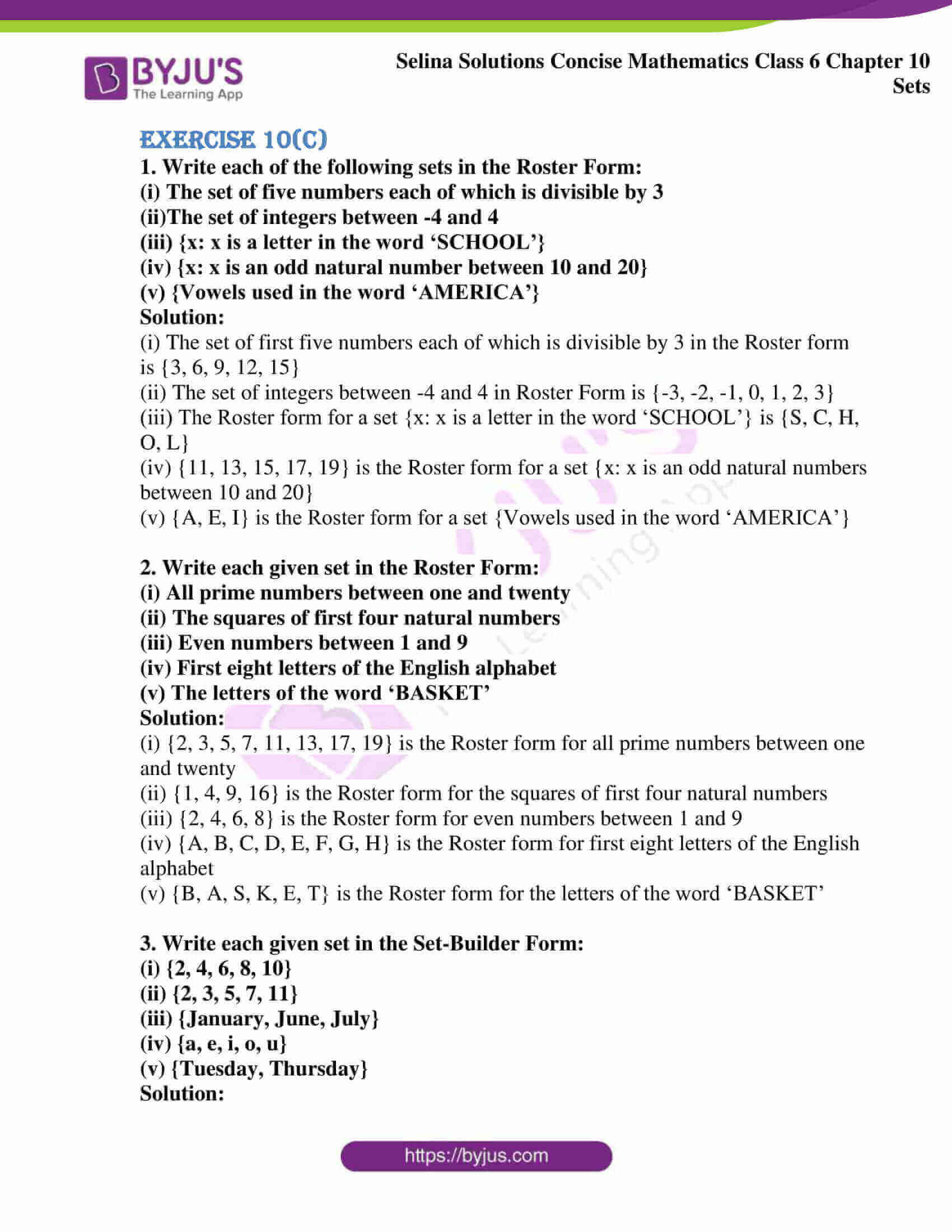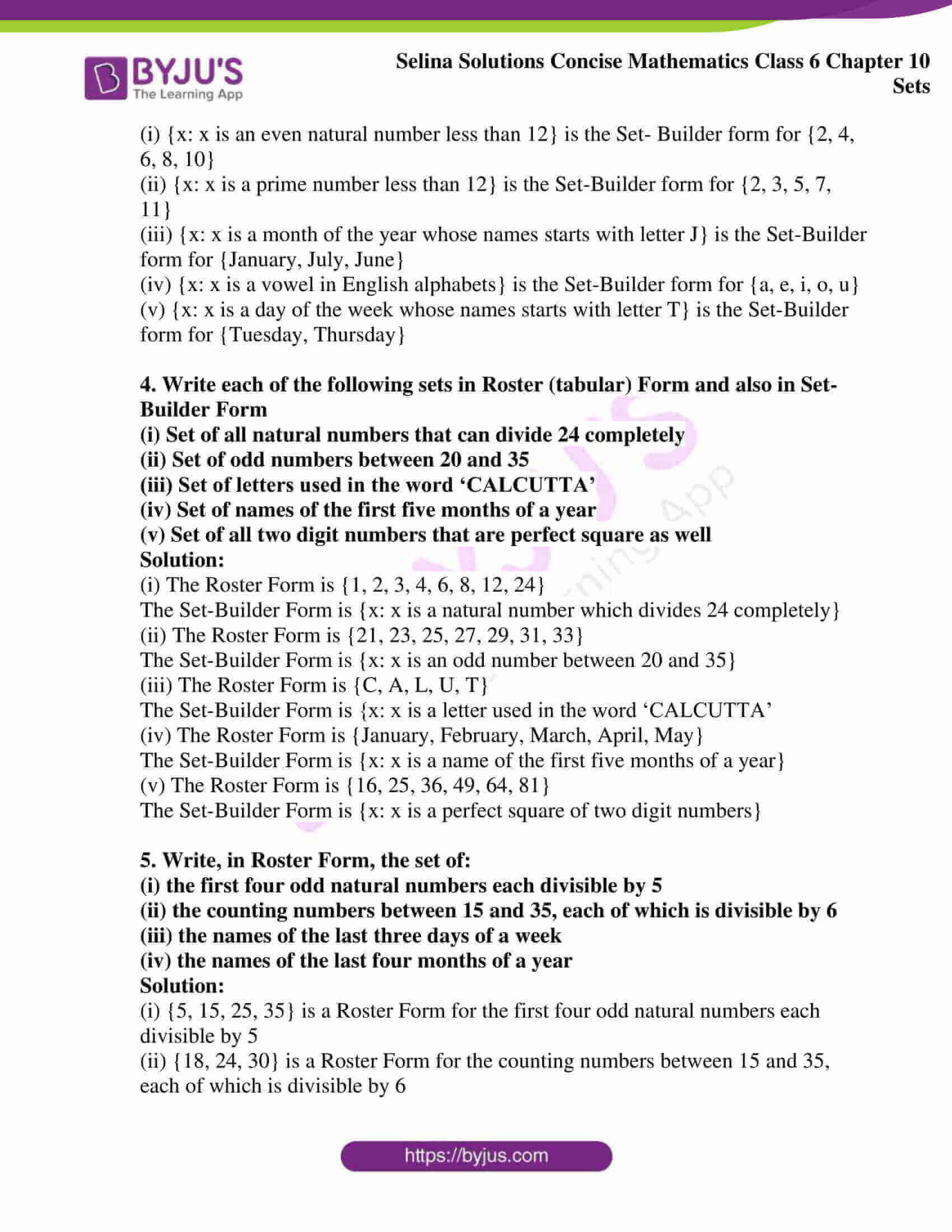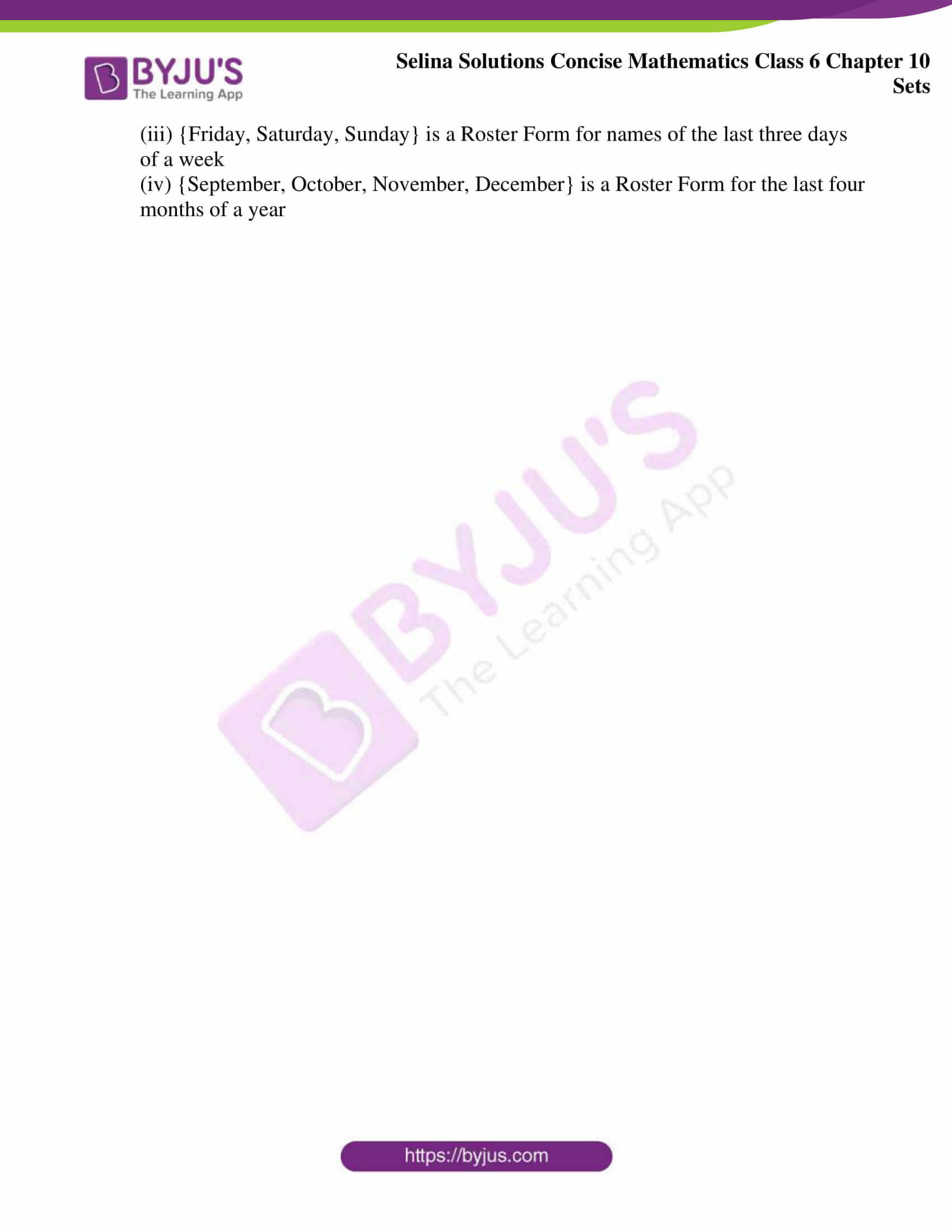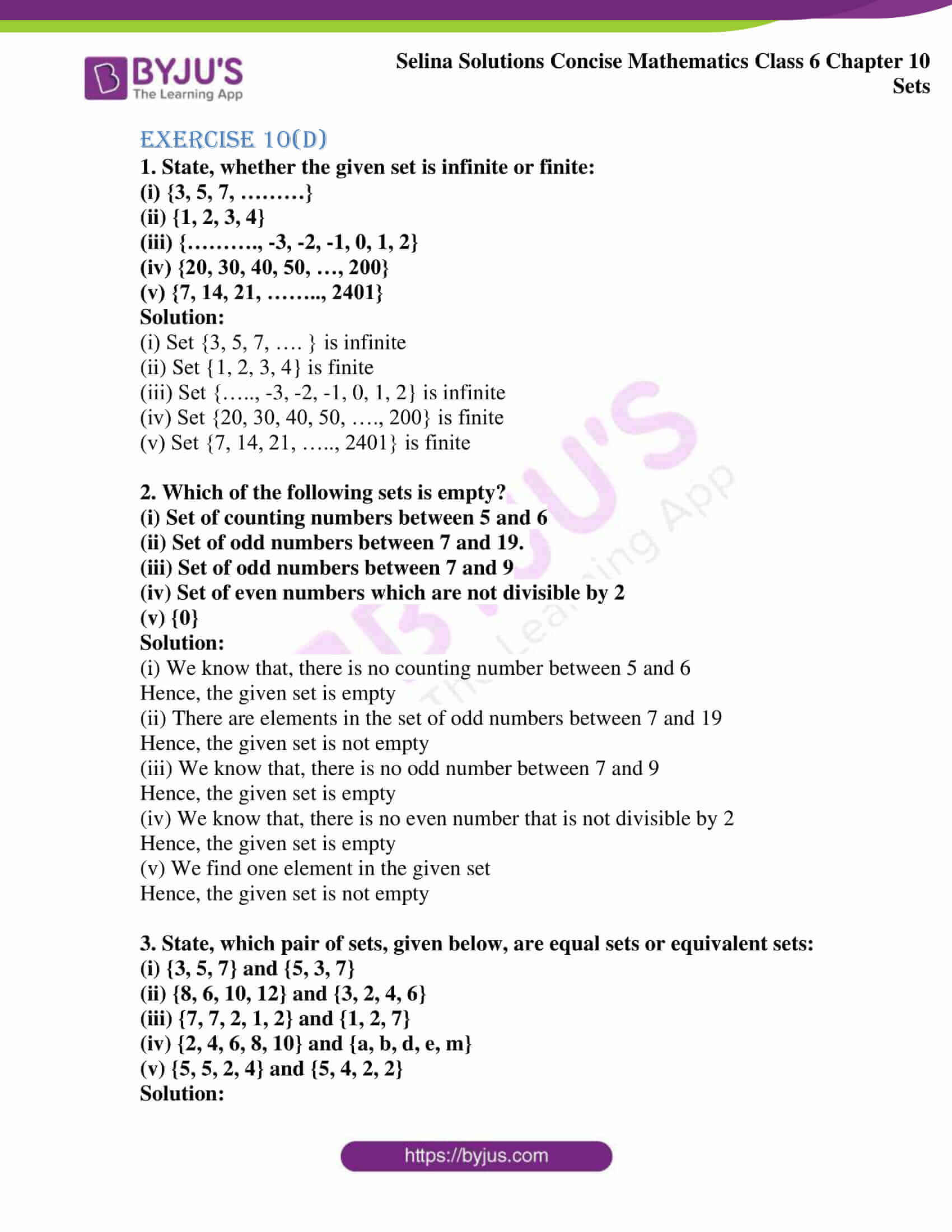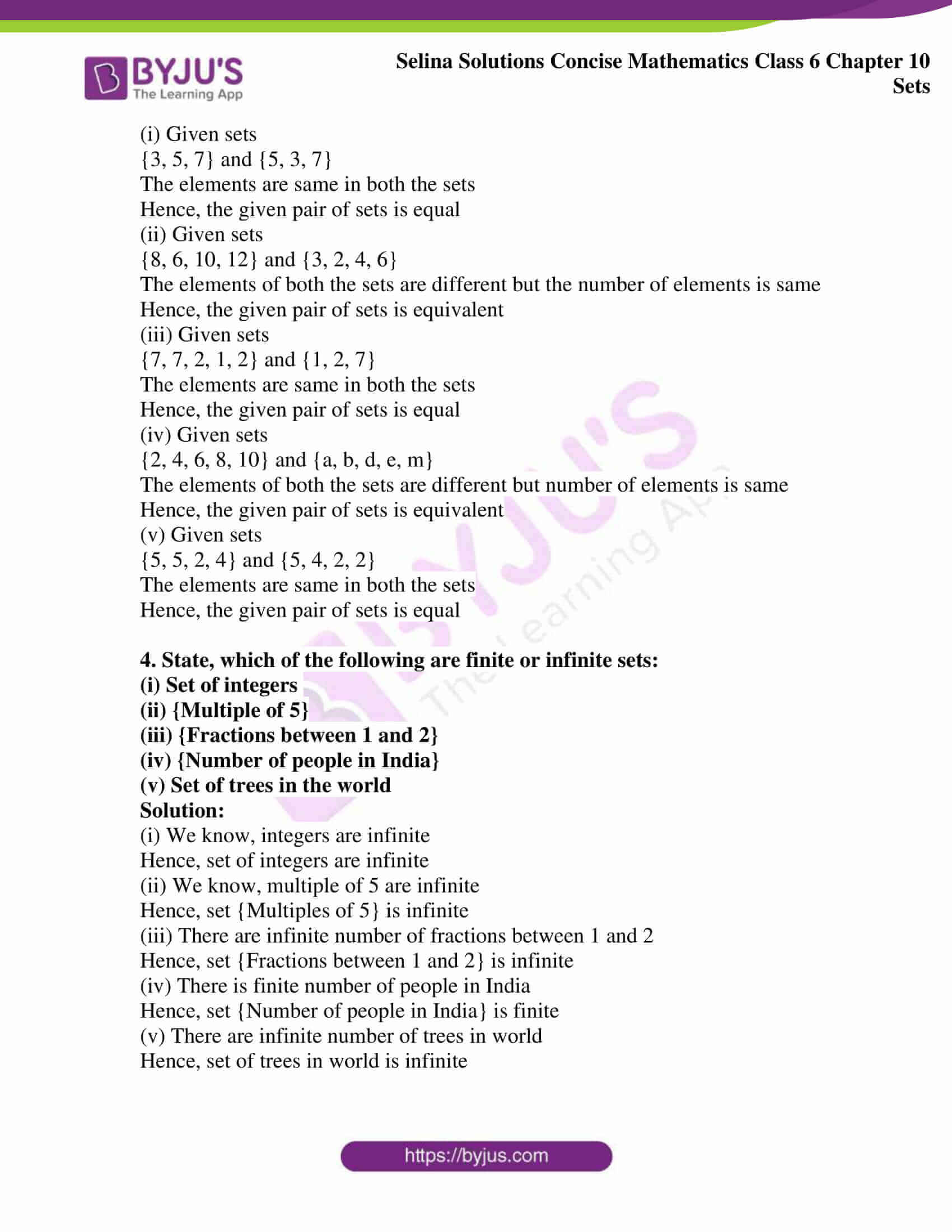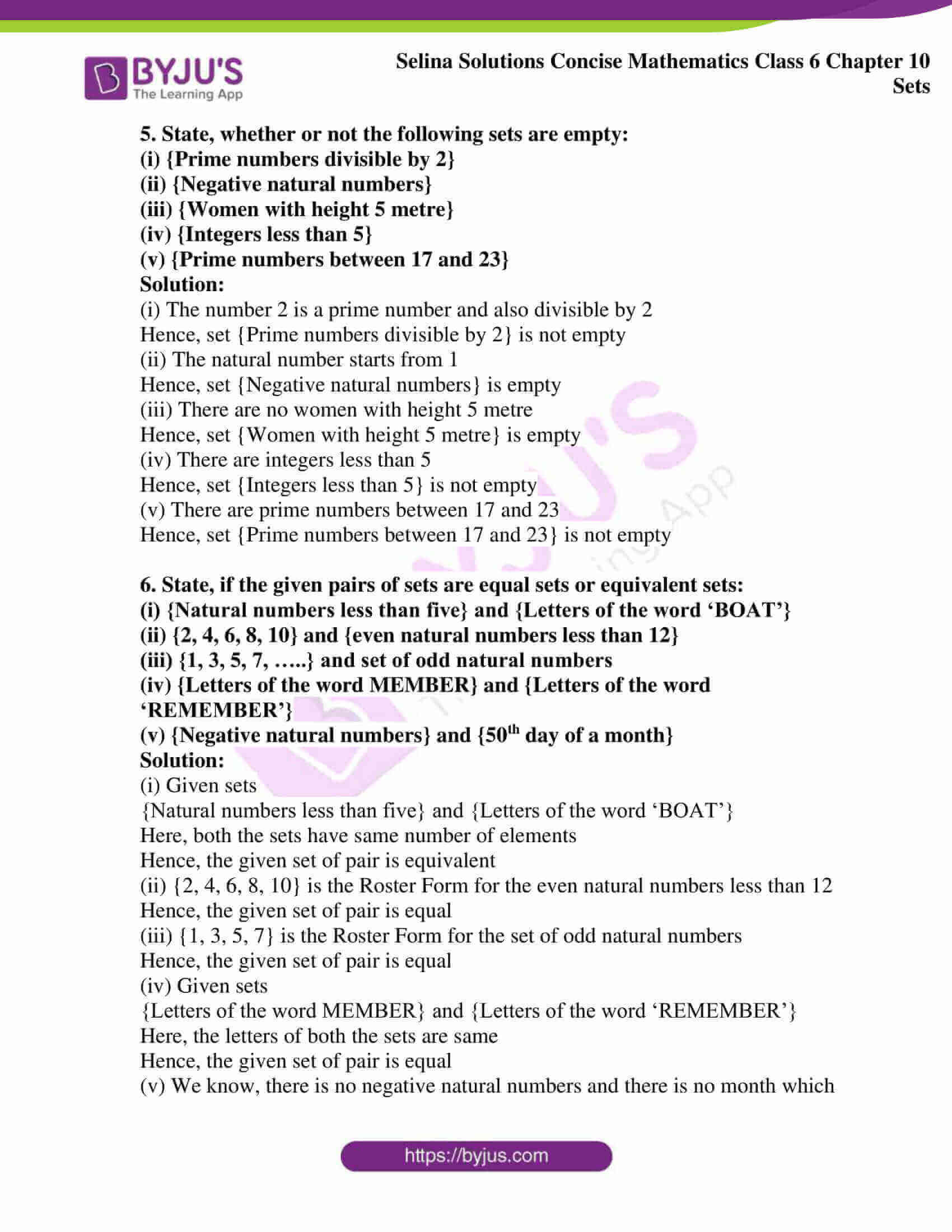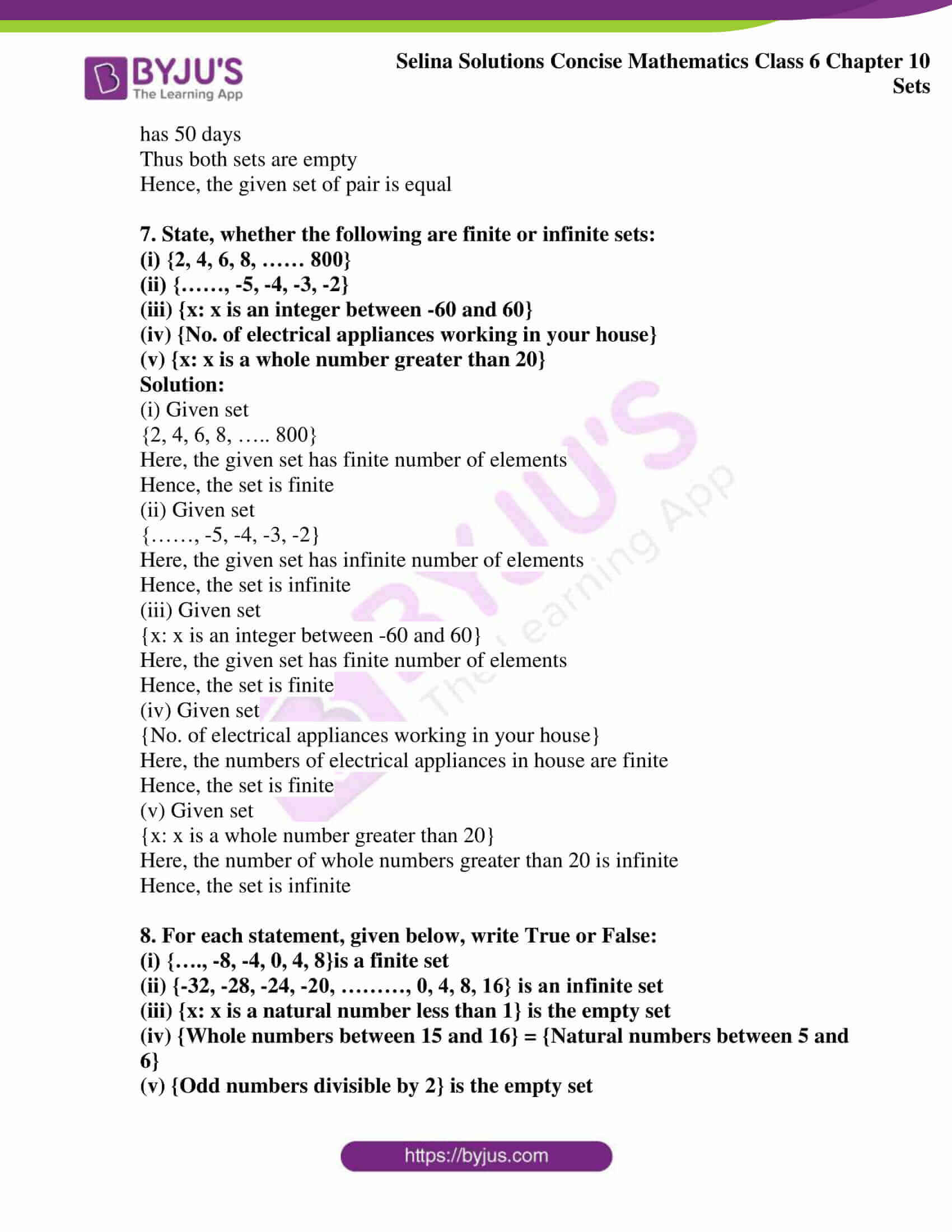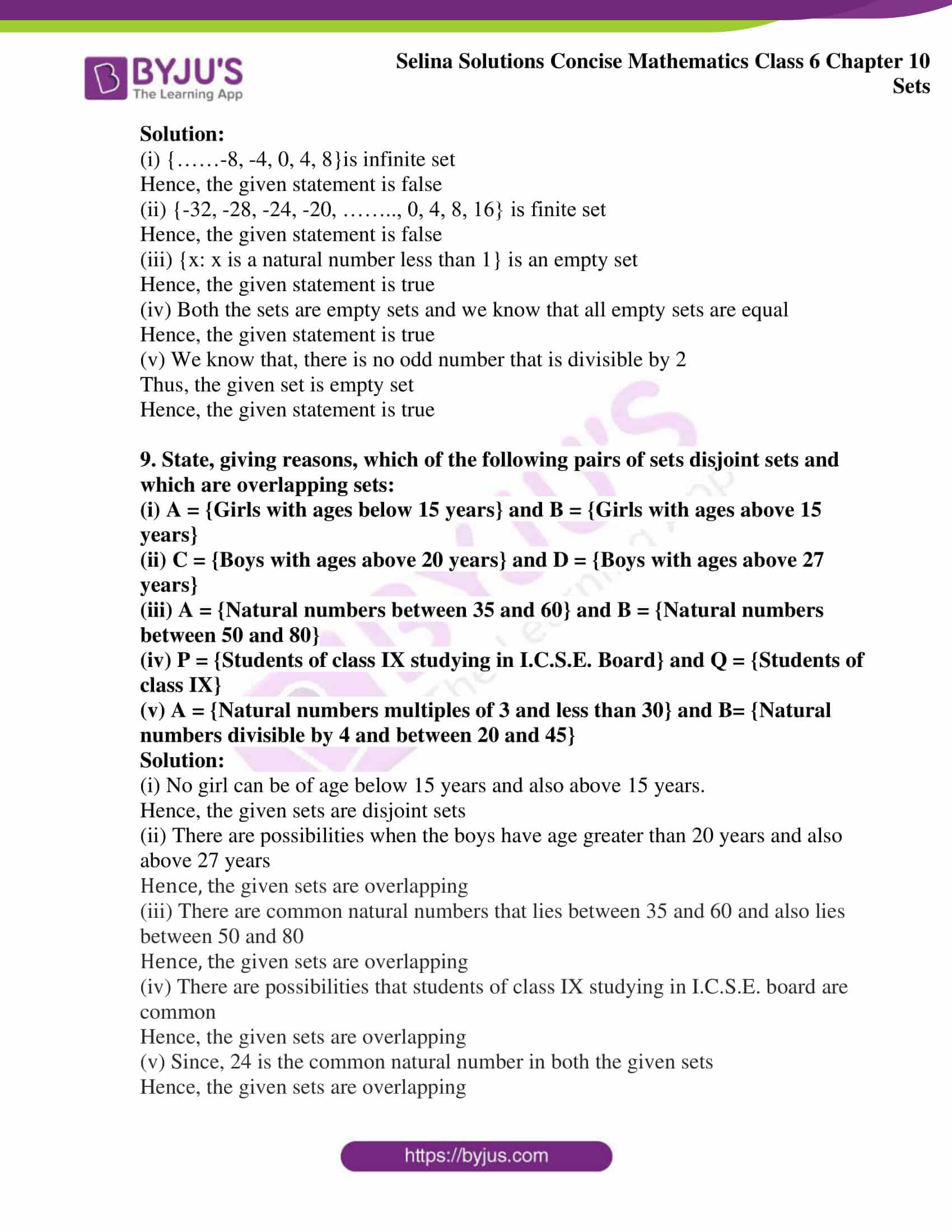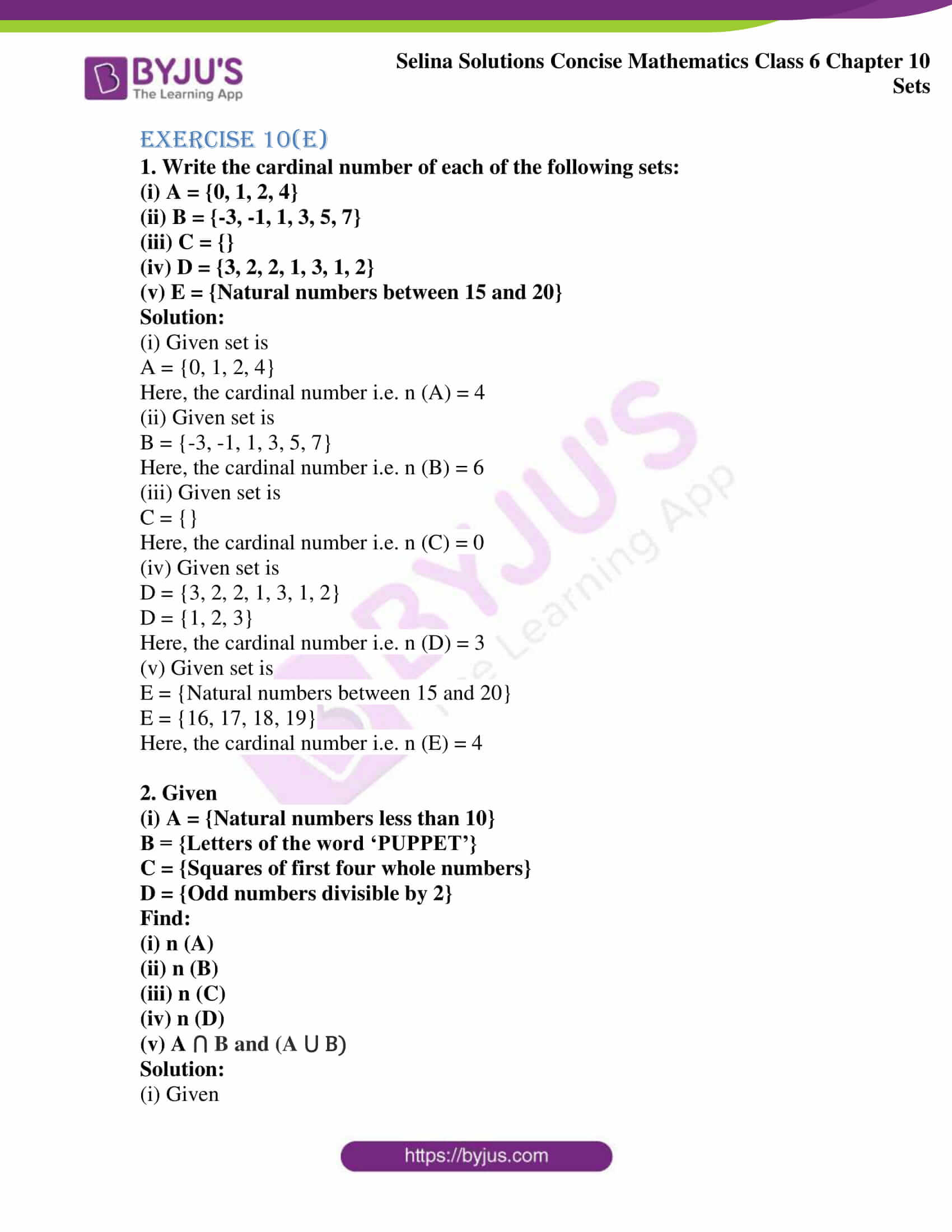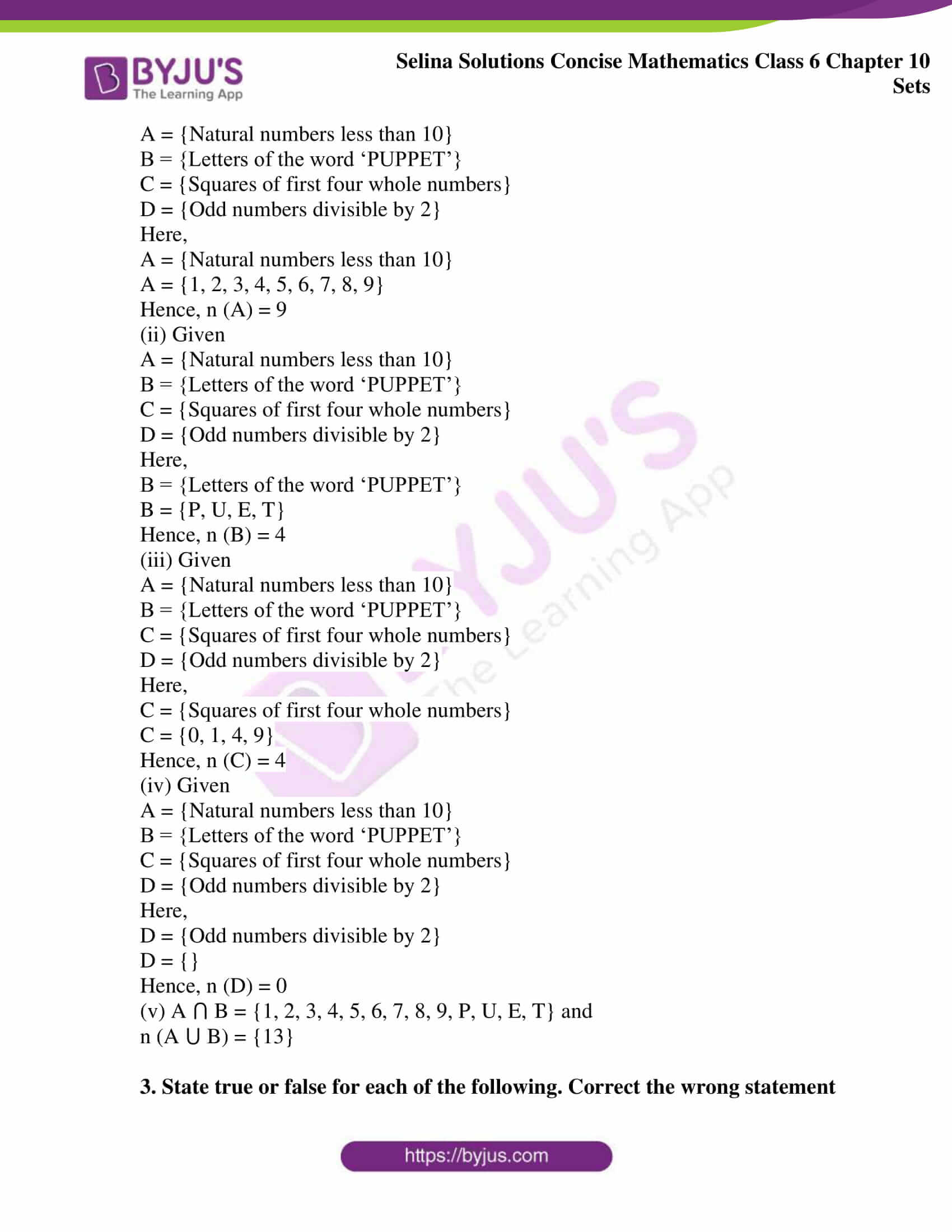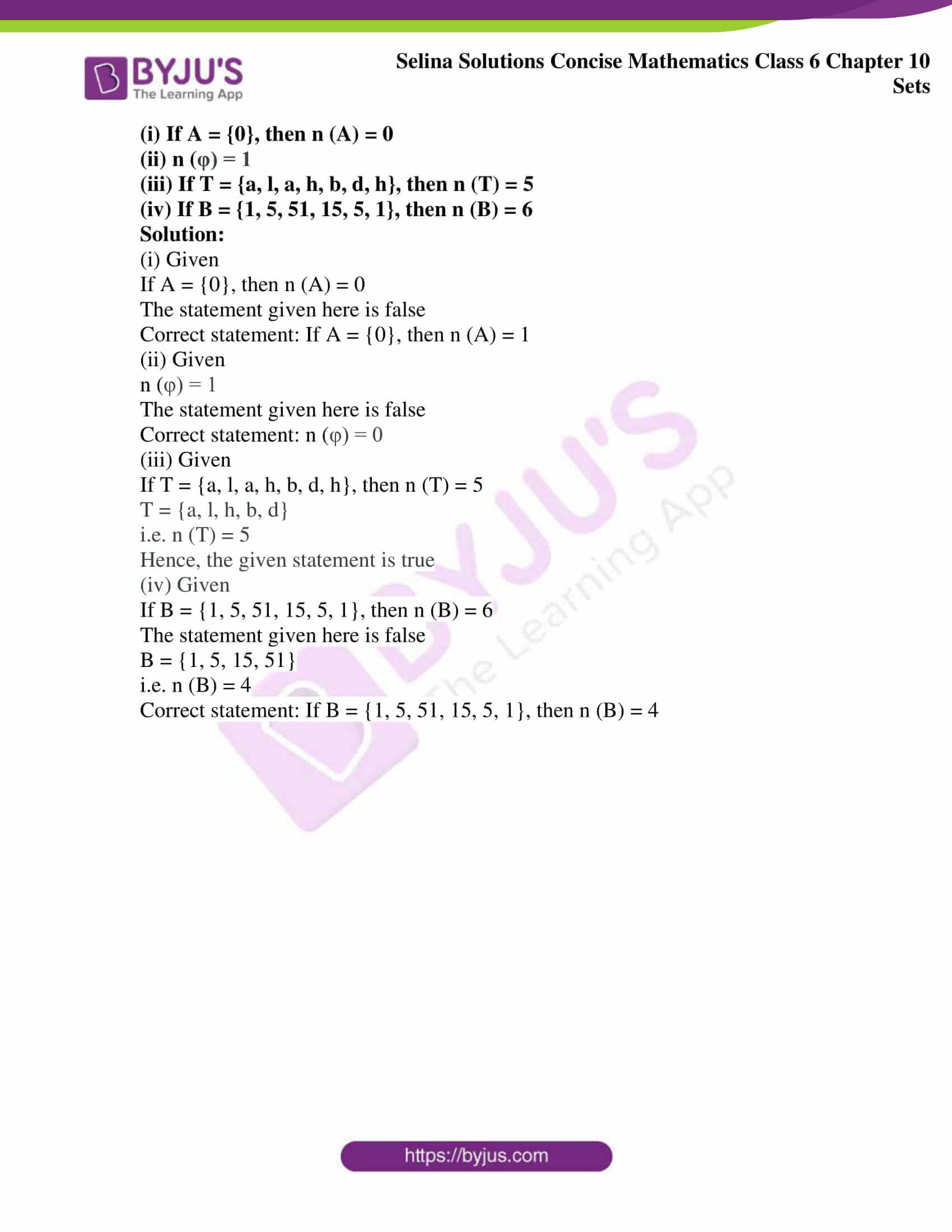## Access Selina Solutions Concise Mathematics Class 6 Chapter 10: Sets

### Exercise 10(A)

1. State whether or not the following elements form a set, if not, give reason:

(i) All easy problems in your text book.

(ii) All three sided figures.

(iii) The first five counting numbers.

(iv) All the tall boys of your class.

(v) The last three days of the week.

(vi) All triangle that are difficult to draw.

(vii) The first three letters of the English alphabet.

(viii) All tasty fruits.

(ix) All clever boys of class 6.

(x) All good schools in Delhi.

(xi) All the girls in your class, whose heights are less than your height.

(xii) All the boys in your class, whose heights are more than your height.

(xiii) All the problems in your Mathematics book, which are difficult for Amit.

Solutions:

(i) No, the given elements do not form a set because some problems may be easy for one person but difficult to some other person. Here the objects are not well defined.

(ii) Yes, the given elements form a set.

(iii) Yes, the given elements form a set.

(iv) No, the objects given here do not form a set because the definition of tall boys is not well defined

(v) Yes, the given elements form a set

(vi) No, the objects given here do not form a set, because it may be difficult to draw a given triangle for a boy but it may be easy for another to draw a same triangle

(vii) Yes, the given elements form a set

(viii) No, the objects given here do not form a set, because a fruit may be tasty for one person but it may not be tasty for another person

(ix) No, the objects given here do not form a set, because cleverness of boys is not well defined

(x) No, the objects given here do not form a set, because all the people do not find the same schools as good as other

(xi) Yes, the given elements form a set

(xii) Yes, the given elements form a set

(xiii) Yes, the given elements form a set

### Exercise 10(B)

1. If set A = {2, 3, 4, 5, 6}, state which of the following statements are true and which are false:

(i) 2 ε A

(ii) 5, 6 ε A

(iii) 3, 4, 7 ε A

(iv) 2, 8 ε A

Solution:

(i) Given

Set A = {2, 3, 4, 5, 6}

Here, element 2 belongs to set A

Hence, the given statement is true

(ii) Given

Set A = {2, 3, 4, 5, 6}

Here, element 5 and 6 belongs to set A

Hence, the given statement is true

(iii) Given

Set A = {2, 3, 4, 5, 6}

Here, element 7 does not belongs to set A

Hence, the given statement is false

(iv) Given

Set A = {2, 3, 4, 5, 6}

Here, element 8 does not belongs to set A

Hence, the given statement is false

2. If set B = {4, 6, 8, 10, 12, 14}. State, which of the following statements is correct and which is wrong:

(i) 5 ε B

(ii) 12 ε B

(iii) 14 ε B

(iv) 9 ε B

(v) B is a set of even numbers between 2 and 16.

(vi) 4, 6 and 10 are die members of the set B. Also, write the wrong statements correctly.

(i) Given

Set B = {4, 6, 8, 10, 12, 14}

Here, element 5 does not belongs to set B

Hence, the given statement is false

(ii) Given

Set B = {4, 6, 8, 10, 12, 14}

Here, element 12 belongs to set B

Hence, the given statement is true

(iii) Given

Set B = {4, 6, 8, 10, 12, 14}

Here, element 14 belongs to set B

Hence, the given statement is true

(iv) Given

Set B = {4, 6, 8, 10, 12, 14}

Here, element 9 does not belongs to set B

Hence, the given statement is false

(v) Given

Set B = {4, 6, 8, 10, 12, 14}

Here, B is a set of even numbers between 2 and 16

Hence, the given statement is true

(vi) Given

Set B = {4, 6, 8, 10, 12, 14}

Here, elements 4, 6, and 10 belongs to set B

Hence, the given statement is true

3. State, whether true of false:

(i) Sets {4, 9, 6, 2} and {6, 2, 4, 9} are not the same.

(ii) Sets {0, 1, 3, 9, 4} and {4, 0, 1, 3, 9} are the same.

(iii) Sets {5, 4} and {5, 4, 4, 5} are not the same.

(iv) Sets {8, 3} and {3, 3, 8} are the same.

(v) Collection of vowels used in the word ‘ALLAHABAD’ forms a set.

(vi) If P is the set of letters in the word ‘ROOP’, then P = (P, O, R)

(vii) If M is the set of letters used in the word ‘MUMBAI’, then M = {M, U, B, A, I}

Solution:

(i) Two sets are said to be equal if they contain same elements

Here, sets {4, 9, 6, 2} and {6, 2, 4, 9} contain same elements

Hence, both sets are same

∴ The given statement is false

(ii) Two sets are said to be equal if they contain same elements

Here, Sets {0, 1, 3, 9, 4} and {4, 0, 1, 3, 9} contain same elements

Hence, both sets are same

∴ The given statement is true

(iii) Two sets are said to be equal if they contain same elements

Here, Sets {5, 4} and {5, 4, 4, 5} contain same elements

Hence, both sets are same

∴ The given statement is false

(iv) Two sets are said to be equal if they contain same elements

Here, sets {8, 3} and {3, 3, 8} contain same elements

Hence, both sets are same

∴ The given statement is true

(v) Given

Collection of vowels used in the word ‘ALLAHABAD’ is a well defined collection

Hence, it form a set

∴ The given statement is true

(vi) Given

A set of letters in the word ‘ROOP’ is {P, O, R}

∴ The given statement is true

(vii) Given

A set of letters in the word ‘MUMBAI’ is {M, U, B, A, I}

∴ The given statement is true

4. Write the set containing:

(i) the first five counting numbers

(ii) the three types of angles

(iii) the three types of triangles

(iv) the members of your family

(v) the first six consonants of the English Alphabet

(vi) the first four vowels of the English Alphabet

(vii) the names of any three Prime – Ministers of India

Solution:

(i) {1, 2, 3, 4, 5} is a set of first five counting numbers

(ii) {right angle, acute angle, obtuse angle} is a set of three types of angles

(iii){right triangle, acute triangle, obtuse triangle} is a set of three types of triangles

(iv) {write the name of your family members} is a set of the members of your family

(v) {b, c, d, f, g, h} is a set of first six consonants of the English Alphabet

(vi) {a, e, i, o} is a set of four vowels of the English Alphabet

(vii) {Narendra Modi, Dr Manmohan Singh, A.B Vajpayee} is a set of three Prime Ministers of India

5. (a) Write the members (elements) of each set given below:

(i) {3, 8, 5, 15, 12, 7}

(ii) {c, m, n, o, s}

(b) Write the sets whose elements are:

(i) 2, 4, 8, 16, 64 and 128

(ii) 3, 5, 15, 45, 75 and 90

Solution:

(a)(i) The members of set {3, 8, 5, 15, 12, 7} are 3, 8, 5, 15, 12 and 7

(ii) The members of set {c, m, n, o, s} are c, m, n, o and s

(b) (i) The set of elements 2, 4, 8, 16, 64 and 128 are {2, 4, 8, 16, 64, 128}

(ii) The set of elements 3, 5, 15, 45, 75 and 90 are {3, 5, 15, 45, 75, 90}

6. (i) Write the set of letters used in the word ‘BHOPAL’.

(ii) Write the set of vowels used in the word ‘BENGAL’

(iii) Write the set of consonants used in the word ‘HONG KONG’

Solution:

(i) {B, H, O, P, A, L} is the set of letters used in the word ‘BHOPAL’

(ii) {A, E} is the set of vowels used in the word ‘BENGAL’

(iii) {H, N, G, K} is the set of consonants used in the word ‘HONG KONG’

### Exercise 10(C)

1. Write each of the following sets in the Roster Form:

(i) The set of five numbers each of which is divisible by 3

(ii)The set of integers between -4 and 4

(iii) {x: x is a letter in the word ‘SCHOOL’}

(iv) {x: x is an odd natural number between 10 and 20}

(v) {Vowels used in the word ‘AMERICA’}

Solution:

(i) The set of first five numbers each of which is divisible by 3 in the Roster form is {3, 6, 9, 12, 15}

(ii) The set of integers between -4 and 4 in Roster Form is {-3, -2, -1, 0, 1, 2, 3}

(iii) The Roster form for a set {x: x is a letter in the word ‘SCHOOL’} is {S, C, H, O, L}

(iv) {11, 13, 15, 17, 19} is the Roster form for a set {x: x is an odd natural numbers between 10 and 20}

(v) {A, E, I} is the Roster form for a set {Vowels used in the word ‘AMERICA’}

2. Write each given set in the Roster Form:

(i) All prime numbers between one and twenty

(ii) The squares of first four natural numbers

(iii) Even numbers between 1 and 9

(iv) First eight letters of the English alphabet

(v) The letters of the word ‘BASKET’

Solution:

(i) {2, 3, 5, 7, 11, 13, 17, 19} is the Roster form for all prime numbers between one and twenty

(ii) {1, 4, 9, 16} is the Roster form for the squares of first four natural numbers

(iii) {2, 4, 6, 8} is the Roster form for even numbers between 1 and 9

(iv) {A, B, C, D, E, F, G, H} is the Roster form for first eight letters of the English alphabet

(v) {B, A, S, K, E, T} is the Roster form for the letters of the word ‘BASKET’

3. Write each given set in the Set-Builder Form:

(i) {2, 4, 6, 8, 10}

(ii) {2, 3, 5, 7, 11}

(iii) {January, June, July}

(iv) {a, e, i, o, u}

(v) {Tuesday, Thursday}

Solution:

(i) {x: x is an even natural number less than 12} is the Set- Builder form for {2, 4, 6, 8, 10}

(ii) {x: x is a prime number less than 12} is the Set-Builder form for {2, 3, 5, 7, 11}

(iii) {x: x is a month of the year whose names starts with letter J} is the Set-Builder form for {January, July, June}

(iv) {x: x is a vowel in English alphabets} is the Set-Builder form for {a, e, i, o, u}

(v) {x: x is a day of the week whose names starts with letter T} is the Set-Builder form for {Tuesday, Thursday}

4. Write each of the following sets in Roster (tabular) Form and also in Set-Builder Form

(i) Set of all natural numbers that can divide 24 completely

(ii) Set of odd numbers between 20 and 35

(iii) Set of letters used in the word ‘CALCUTTA’

(iv) Set of names of the first five months of a year

(v) Set of all two digit numbers that are perfect square as well

Solution:

(i) The Roster Form is {1, 2, 3, 4, 6, 8, 12, 24}

The Set-Builder Form is {x: x is a natural number which divides 24 completely}

(ii) The Roster Form is {21, 23, 25, 27, 29, 31, 33}

The Set-Builder Form is {x: x is an odd number between 20 and 35}

(iii) The Roster Form is {C, A, L, U, T}

The Set-Builder Form is {x: x is a letter used in the word ‘CALCUTTA’

(iv) The Roster Form is {January, February, March, April, May}

The Set-Builder Form is {x: x is a name of the first five months of a year}

(v) The Roster Form is {16, 25, 36, 49, 64, 81}

The Set-Builder Form is {x: x is a perfect square of two digit numbers}

5. Write, in Roster Form, the set of:

(i) the first four odd natural numbers each divisible by 5

(ii) the counting numbers between 15 and 35, each of which is divisible by 6

(iii) the names of the last three days of a week

(iv) the names of the last four months of a year

Solution:

(i) {5, 15, 25, 35} is a Roster Form for the first four odd natural numbers each divisible by 5

(ii) {18, 24, 30} is a Roster Form for the counting numbers between 15 and 35, each of which is divisible by 6

(iii) {Friday, Saturday, Sunday} is a Roster Form for names of the last three days of a week

(iv) {September, October, November, December} is a Roster Form for the last four months of a year

### Exercise 10(D)

1. State, whether the given set is infinite or finite:

(i) {3, 5, 7, ………}

(ii) {1, 2, 3, 4}

(iii) {………., -3, -2, -1, 0, 1, 2}

(iv) {20, 30, 40, 50, …, 200}

(v) {7, 14, 21, …….., 2401}

Solution:

(i) Set {3, 5, 7, …. } is infinite

(ii) Set {1, 2, 3, 4} is finite

(iii) Set {….., -3, -2, -1, 0, 1, 2} is infinite

(iv) Set {20, 30, 40, 50, …., 200} is finite

(v) Set {7, 14, 21, ….., 2401} is finite

2. Which of the following sets is empty?

(i) Set of counting numbers between 5 and 6

(ii) Set of odd numbers between 7 and 19.

(iii) Set of odd numbers between 7 and 9

(iv) Set of even numbers which are not divisible by 2

(v) {0}

Solution:

(i) We know that, there is no counting number between 5 and 6

Hence, the given set is empty

(ii) There are elements in the set of odd numbers between 7 and 19

Hence, the given set is not empty

(iii) We know that, there is no odd number between 7 and 9

Hence, the given set is empty

(iv) We know that, there is no even number that is not divisible by 2

Hence, the given set is empty

(v) We find one element in the given set

Hence, the given set is not empty

3. State, which pair of sets, given below, are equal sets or equivalent sets:

(i) {3, 5, 7} and {5, 3, 7}

(ii) {8, 6, 10, 12} and {3, 2, 4, 6}

(iii) {7, 7, 2, 1, 2} and {1, 2, 7}

(iv) {2, 4, 6, 8, 10} and {a, b, d, e, m}

(v) {5, 5, 2, 4} and {5, 4, 2, 2}

Solution:

(i) Given sets

{3, 5, 7} and {5, 3, 7}

The elements are same in both the sets

Hence, the given pair of sets is equal

(ii) Given sets

{8, 6, 10, 12} and {3, 2, 4, 6}

The elements of both the sets are different but the number of elements is same

Hence, the given pair of sets is equivalent

(iii) Given sets

{7, 7, 2, 1, 2} and {1, 2, 7}

The elements are same in both the sets

Hence, the given pair of sets is equal

(iv) Given sets

{2, 4, 6, 8, 10} and {a, b, d, e, m}

The elements of both the sets are different but number of elements is same

Hence, the given pair of sets is equivalent

(v) Given sets

{5, 5, 2, 4} and {5, 4, 2, 2}

The elements are same in both the sets

Hence, the given pair of sets is equal

4. State, which of the following are finite or infinite sets:

(i) Set of integers

(ii) {Multiple of 5}

(iii) {Fractions between 1 and 2}

(iv) {Number of people in India}

(v) Set of trees in the world

Solution:

(i) We know, integers are infinite

Hence, set of integers are infinite

(ii) We know, multiple of 5 are infinite

Hence, set {Multiples of 5} is infinite

(iii) There are infinite number of fractions between 1 and 2

Hence, set {Fractions between 1 and 2} is infinite

(iv) There is finite number of people in India

Hence, set {Number of people in India} is finite

(v) There are infinite number of trees in world

Hence, set of trees in world is infinite

5. State, whether or not the following sets are empty:

(i) {Prime numbers divisible by 2}

(ii) {Negative natural numbers}

(iii) {Women with height 5 metre}

(iv) {Integers less than 5}

(v) {Prime numbers between 17 and 23}

Solution:

(i) The number 2 is a prime number and also divisible by 2

Hence, set {Prime numbers divisible by 2} is not empty

(ii) The natural number starts from 1

Hence, set {Negative natural numbers} is empty

(iii) There are no women with height 5 metre

Hence, set {Women with height 5 metre} is empty

(iv) There are integers less than 5

Hence, set {Integers less than 5} is not empty

(v) There are prime numbers between 17 and 23

Hence, set {Prime numbers between 17 and 23} is not empty

6. State, if the given pairs of sets are equal sets or equivalent sets:

(i) {Natural numbers less than five} and {Letters of the word ‘BOAT’}

(ii) {2, 4, 6, 8, 10} and {even natural numbers less than 12}

(iii) {1, 3, 5, 7, …..} and set of odd natural numbers

(iv) {Letters of the word MEMBER} and {Letters of the word ‘REMEMBER’}

(v) {Negative natural numbers} and {50th day of a month}

Solution:

(i) Given sets

{Natural numbers less than five} and {Letters of the word ‘BOAT’}

Here, both the sets have same number of elements

Hence, the given set of pair is equivalent

(ii) {2, 4, 6, 8, 10} is the Roster Form for the even natural numbers less than 12

Hence, the given set of pair is equal

(iii) {1, 3, 5, 7} is the Roster Form for the set of odd natural numbers

Hence, the given set of pair is equal

(iv) Given sets

{Letters of the word MEMBER} and {Letters of the word ‘REMEMBER’}

Here, the letters of both the sets are same

Hence, the given set of pair is equal

(v) We know, there is no negative natural numbers and there is no month which has 50 days

Thus both sets are empty

Hence, the given set of pair is equal

7. State, whether the following are finite or infinite sets:

(i) {2, 4, 6, 8, …… 800}

(ii) {……, -5, -4, -3, -2}

(iii) {x: x is an integer between -60 and 60}

(iv) {No. of electrical appliances working in your house}

(v) {x: x is a whole number greater than 20}

Solution:

(i) Given set

{2, 4, 6, 8, ….. 800}

Here, the given set has finite number of elements

Hence, the set is finite

(ii) Given set

{……, -5, -4, -3, -2}

Here, the given set has infinite number of elements

Hence, the set is infinite

(iii) Given set

{x: x is an integer between -60 and 60}

Here, the given set has finite number of elements

Hence, the set is finite

(iv) Given set

{No. of electrical appliances working in your house}

Here, the numbers of electrical appliances in house are finite

Hence, the set is finite

(v) Given set

{x: x is a whole number greater than 20}

Here, the number of whole numbers greater than 20 is infinite

Hence, the set is infinite

8. For each statement, given below, write True or False:

(i) {…., -8, -4, 0, 4, 8}is a finite set

(ii) {-32, -28, -24, -20, ………, 0, 4, 8, 16} is an infinite set

(iii) {x: x is a natural number less than 1} is the empty set

(iv) {Whole numbers between 15 and 16} = {Natural numbers between 5 and 6}

(v) {Odd numbers divisible by 2} is the empty set

Solution:

(i) {……-8, -4, 0, 4, 8}is infinite set

Hence, the given statement is false

(ii) {-32, -28, -24, -20, …….., 0, 4, 8, 16} is finite set

Hence, the given statement is false

(iii) {x: x is a natural number less than 1} is an empty set

Hence, the given statement is true

(iv) Both the sets are empty sets and we know that all empty sets are equal

Hence, the given statement is true

(v) We know that, there is no odd number that is divisible by 2

Thus, the given set is empty set

Hence, the given statement is true

9. State, giving reasons, which of the following pairs of sets disjoint sets and which are overlapping sets:

(i) A = {Girls with ages below 15 years} and B = {Girls with ages above 15 years}

(ii) C = {Boys with ages above 20 years} and D = {Boys with ages above 27 years}

(iii) A = {Natural numbers between 35 and 60} and B = {Natural numbers between 50 and 80}

(iv) P = {Students of class IX studying in I.C.S.E. Board} and Q = {Students of class IX}

(v) A = {Natural numbers multiples of 3 and less than 30} and B= {Natural numbers divisible by 4 and between 20 and 45}

Solution:

(i) No girl can be of age below 15 years and also above 15 years.

Hence, the given sets are disjoint sets

(ii) There are possibilities when the boys have age greater than 20 years and also above 27 years

Hence, the given sets are overlapping

(iii) There are common natural numbers that lies between 35 and 60 and also lies between 50 and 80

Hence, the given sets are overlapping

(iv) There are possibilities that students of class IX studying in I.C.S.E. board are common

Hence, the given sets are overlapping

(v) Since, 24 is the common natural number in both the given sets

Hence, the given sets are overlapping

### Exercise 10(E)

1. Write the cardinal number of each of the following sets:

(i) A = {0, 1, 2, 4}

(ii) B = {-3, -1, 1, 3, 5, 7}

(iii) C = {}

(iv) D = {3, 2, 2, 1, 3, 1, 2}

(v) E = {Natural numbers between 15 and 20}

Solution:

(i) Given set is

A = {0, 1, 2, 4}

Here, the cardinal number i.e. n (A) = 4

(ii) Given set is

B = {-3, -1, 1, 3, 5, 7}

Here, the cardinal number i.e. n (B) = 6

(iii) Given set is

C = {}

Here, the cardinal number i.e. n (C) = 0

(iv) Given set is

D = {3, 2, 2, 1, 3, 1, 2}

D = {1, 2, 3}

Here, the cardinal number i.e. n (D) = 3

(v) Given set is

E = {Natural numbers between 15 and 20}

E = {16, 17, 18, 19}

Here, the cardinal number i.e. n (E) = 4

2. Given

(i) A = {Natural numbers less than 10}

B = {Letters of the word ‘PUPPET’}

C = {Squares of first four whole numbers}

D = {Odd numbers divisible by 2}

Find:

(i) n (A)

(ii) n (B)

(iii) n (C)

(iv) n (D)

(v) A ⋂ B and (A ⋃ B)

Solution:

(i) Given

A = {Natural numbers less than 10}

B = {Letters of the word ‘PUPPET’}

C = {Squares of first four whole numbers}

D = {Odd numbers divisible by 2}

Here,

A = {Natural numbers less than 10}

A = {1, 2, 3, 4, 5, 6, 7, 8, 9}

Hence, n (A) = 9

(ii) Given

A = {Natural numbers less than 10}

B = {Letters of the word ‘PUPPET’}

C = {Squares of first four whole numbers}

D = {Odd numbers divisible by 2}

Here,

B = {Letters of the word ‘PUPPET’}

B = {P, U, E, T}

Hence, n (B) = 4

(iii) Given

A = {Natural numbers less than 10}

B = {Letters of the word ‘PUPPET’}

C = {Squares of first four whole numbers}

D = {Odd numbers divisible by 2}

Here,

C = {Squares of first four whole numbers}

C = {0, 1, 4, 9}

Hence, n (C) = 4

(iv) Given

A = {Natural numbers less than 10}

B = {Letters of the word ‘PUPPET’}

C = {Squares of first four whole numbers}

D = {Odd numbers divisible by 2}

Here,

D = {Odd numbers divisible by 2}

D = {}

Hence, n (D) = 0

(v) A ⋂ B = {1, 2, 3, 4, 5, 6, 7, 8, 9, P, U, E, T} and

n (A ⋃ B) = {13}

3. State true or false for each of the following. Correct the wrong statement

(i) If A = {0}, then n (A) = 0

(ii) n (φ) = 1

(iii) If T = {a, l, a, h, b, d, h}, then n (T) = 5

(iv) If B = {1, 5, 51, 15, 5, 1}, then n (B) = 6

Solution:

(i) Given

If A = {0}, then n (A) = 0

The statement given here is false

Correct statement: If A = {0}, then n (A) = 1

(ii) Given

n (φ) = 1

The statement given here is false

Correct statement: n (φ) = 0

(iii) Given

If T = {a, l, a, h, b, d, h}, then n (T) = 5

T = {a, l, h, b, d}

i.e. n (T) = 5

Hence, the given statement is true

(iv) Given

If B = {1, 5, 51, 15, 5, 1}, then n (B) = 6

The statement given here is false

B = {1, 5, 15, 51}

i.e. n (B) = 4

Correct statement: If B = {1, 5, 51, 15, 5, 1}, then n (B) = 4

### Exercises of Selina Solutions Concise Mathematics Class 6 Chapter 10: Sets

Exercise 10(A) Solutions

Exercise 10(B) Solutions

Exercise 10(C) Solutions

Exercise 10(D) Solutions

Exercise 10(E) Solutions